# Newton’s Laws of Motion

Newton's three laws of motion help explain everyday phenomena that we see in the world around us. Engineers use Newton's laws to navigate space travel, simulate vehicle collisions to improve safety measures and design simple devices like scissors!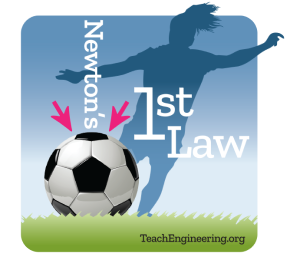A body at rest will stay at rest, and a body in motion will stay in motion, until acted upon by an outside force.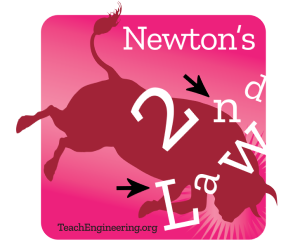Force is equal to mass times acceleration. For an object with a constant mass (m), this law states that the force (F) is the product of an object’s mass and its acceleration (a): F = ma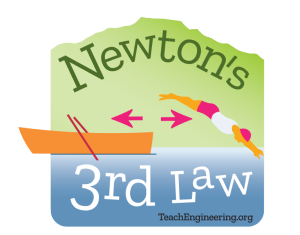For every action there is an equal and opposite reaction. This law is also known as the law of action and reaction.

Engineers apply Newton’s laws of motion in a wide range of designs involving stationary and moving objects, including structures such as bridges, vehicles such as rockets and aircrafts, and other commonly-used objects like seat belts, door knobs and medicine delivery systems.

Engineers must fully understand the workings of the natural physical laws so they can design objects that perform as expected and are safe to use.

Check out the curricula below to give students a push into making sense of everyday phenomena associated with Newton’s laws of motion!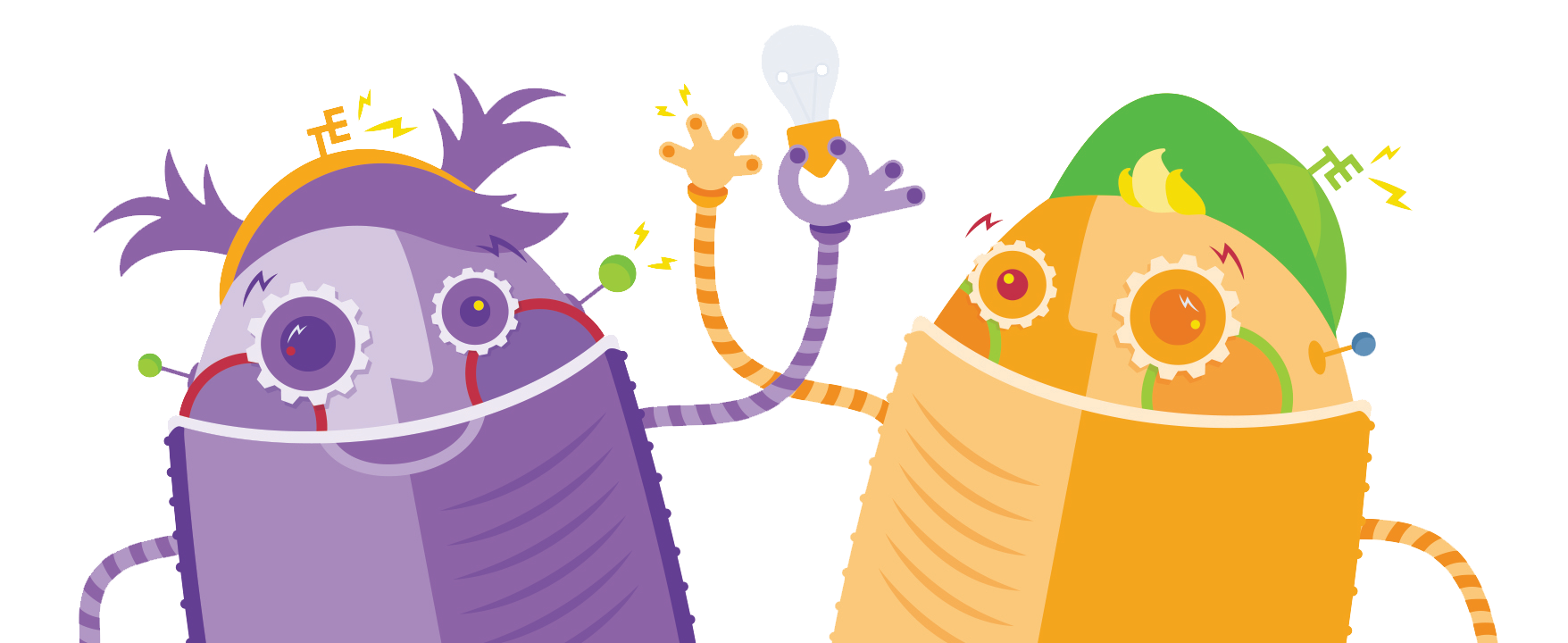Newton’s Laws of Motion CurriculaThe engaging resources from TeachEngineering, featured here by grade band, exemplify Newton’s Laws of Motion curricula.

• Coming Soon!

• Newton Gets Me Moving
Newton Gets Me Moving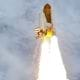Students explore motion, rockets and rocket motion while assisting Spacewoman Tess, Spaceman Rohan and Maya in their explorations. First they learn some basic facts about vehicles, rockets and why we use them. Then, they discover that the motion of all objects—including the flight of a rocket and mo...

• Newton Rocket Car
Newton Rocket Car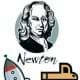Through the use of small wooden cars, this activity demonstrates Newton's third law of motion—which states that every action has an equal and opposite reaction. The "Newton rocket cars" that students put together show how action/reaction works and how the mass of a moving object affects the accelera...

• Rocket Power
Rocket Power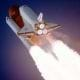By making and testing simple balloon rockets, students acquire a basic understanding of Newton's third law of motion as it applies to rockets. Using balloons, string, straws and tape, they see how rockets are propelled by expelling gases, and test their rockets in horizontal and incline conditions. ...

• Using Thrust, Weight & Control: Rocket Me into Space
Using Thrust, Weight & Control: Rocket Me into SpaceThrough the continuing storyline of the Rockets unit, this lesson looks more closely at Spaceman Rohan, Spacewoman Tess, their daughter Maya, and their challenges with getting to space, setting up satellites, and exploring uncharted waters via a canoe. Students are introduced to the ideas of thrust,...

• Pop Rockets
Pop Rockets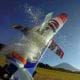Students design and build paper rockets around film canisters, which serve as engines. An antacid tablet and water are put into each canister, reacting to form carbon dioxide gas, and acting as the pop rocket's propellant. With the lid snapped on, the continuous creation of gas causes pressure to bu...

• Physics Tug of War
Physics Tug of War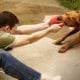Students learn about Newton's second law of motion: force = mass x acceleration. In a tug-of-war experimental setup using paperclips, rubber bands and text books, they collect data and make calculations, seeing that the force required to move a book is proportional to the weight of the book.

• Sliding Textbooks
Sliding Textbooks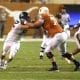In the culminating activity of the unit, students explore and apply their knowledge of forces, friction, acceleration and gravity in a two-part experiment. First, student groups measure the average acceleration of a textbook pulled along a table by varying weights (with optional extensions, such as ...

• What Is Newton's First Law?
What Is Newton's First Law?Students are introduced to the concepts of force, inertia and Newton's first law of motion: objects at rest stay at rest and objects in motion stay in motion unless acted upon by an unbalanced force. Students learn the difference between speed, velocity and acceleration, and come to see that the cha...

• What Is Newton's Second Law?
What Is Newton's Second Law?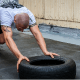Students are introduced to Newton's second law of motion: force = mass x acceleration. Both the mathematical equation and physical examples are discussed, including Atwood's Machine to illustrate the principle. Students come to understand that an object's acceleration depends on its mass and the str...

• What Is Newton's Third Law?
What Is Newton's Third Law?Students are introduced to Newton's third law of motion: For every action, there is an equal and opposite reaction. They practice identifying action-reaction force pairs for a variety of real-world examples, and draw and explain simplified free-body diagram vectors (arrows) of force, velocity and ac...

• Rocket Launch Time: Flying with Style
Rocket Launch Time: Flying with StyleAs students begin to understand the physics behind thrust, drag and gravity and how these relate these to Newton's three laws of motion, groups assemble and launch the rockets that they designed in the associated lesson. The height of the rockets, after constructed and launched, are measured and com...

• Rocketry Calculations: Houston, We Have a Problem!
Rocketry Calculations: Houston, We Have a Problem!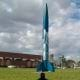They learn about design issues faced by aerospace engineers when trying to launch rocketships or satellites in order to land them safely—in the ocean, for example.

• Rockets!
Rockets!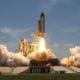Students are introduced to statics and dynamics, free-body diagrams, combustion and thermodynamics to gain an understanding of the forces needed to lift rockets off the ground. They learn that thrust force is needed to launch rockets into space and the energy for thrust is stored as chemical energy ...

• When Should I Drink My Hot Chocolate?
When Should I Drink My Hot Chocolate?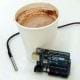Students act as food science engineers as they explore and apply their understanding of cooling rate and specific heat capacity by completing two separate, but interconnected, tasks. They collect and graph data to create a mathematical model that represents the cooling rate, and use an exponential ...

• Sliders (for High School)
Sliders (for High School)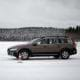In this hands-on activity, students learn about two types of friction — static and kinetic — and the equation that governs them. They also measure the coefficient of static friction and the coefficient of kinetic friction experimentally.

More Popular Topics to explore
Free K-12 standards-aligned STEM curriculum for educators everywhere.
Find more at TeachEngineering.org